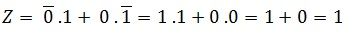# Exclusive OR Gate – EXOR Gate

The EXOR gate is used extensively in digital data processing circuits and is known as Exclusive-OR-gate. The EXOR gate has a high output only when an odd number of inputs are high. For the two input EXOR gates, the output will be high when the set of input is either 01 or 10.

The XOR gate is used in the adder, subtractor and controlled inverter circuit. It is also used in the computers for implementing the binary addition.The logic symbol of the EXOR gate is shown above. The output of an exclusive OR gate will be low for the set of input is either 00 or 11. Therefore, the gate can be used as a Comparator where it gives high output if both the inputs are not equal.

The logic circuit of the gate is shown below:The truth table for this logic gate for all set of the inputs 00, 01, 10 or 11 is shown below:

ABZ
000
011
101
110

For AB: 00For AB: 01For AB: 10For AB: 11From the truth table, it is analysed that the output is high when A or B is high, but it is not high when both are high. That’s why this logic circuit is known as an exclusive-OR gate.

Thus, the inputs must be different to get high output from the two input exclusive OR gate.